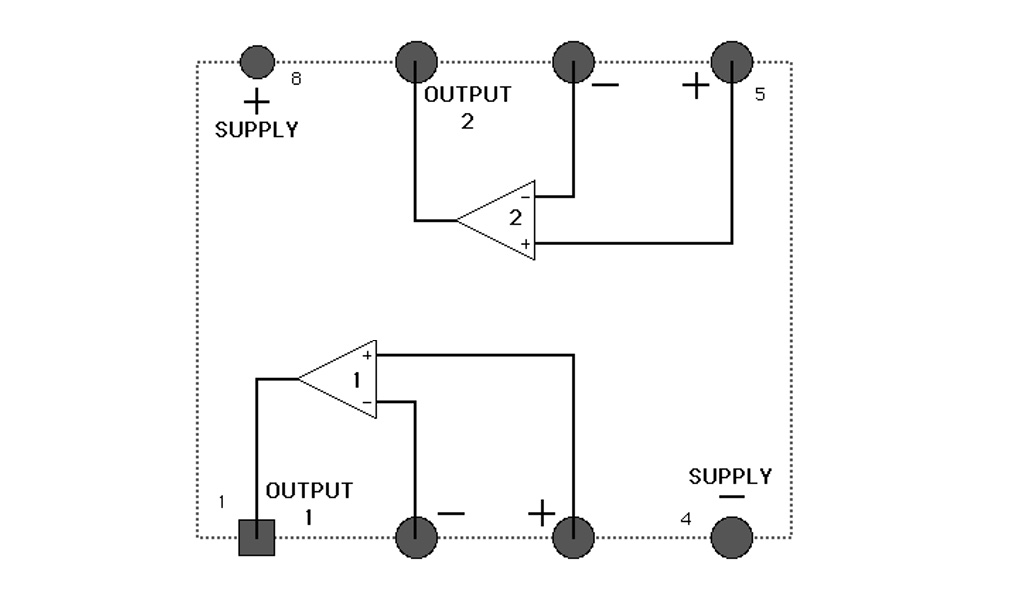## Voltage Comparator - LM393A voltage comparator, as its name implies, compares two voltage signals at both inputs (+ & -) and determines which one is greater. The voltage comparator is one of the simplest example of how logic can be applied in a circuit.

Being able to compare two voltage inputs is very handy in certain situations. Some sensitive circuits might not be able to handle a certain level of voltage. A Comparator can be utilised to check an input voltage with the threshold voltage to ensure that the input won't damage the circuit. Another use of Comparators is to check on the condition of the external environment. For example, a comparator can be utilised to check if the moisture of the soil is too low through checking the conductivity of the soil.

In essence, the LM393 chip is a dual-comparator, meaning that it can perform such task with two different signals. Here's how it works:

• Current WILL flow through the Open Collector (Output) when the voltage at the PLUS input is lower than the voltage at the MINUS input.
• Current WILL NOT flow through the Open Collector (Output) when the voltage at the PLUS input is higher than the voltage at the MINUS input.

Open collector type outputs can only sink current. This means that there is no true output from a collector but rather that a current or voltage must be supplied to the output terminal from a source elsewhere in the larger circuit.

Let's see how it all works in more details.